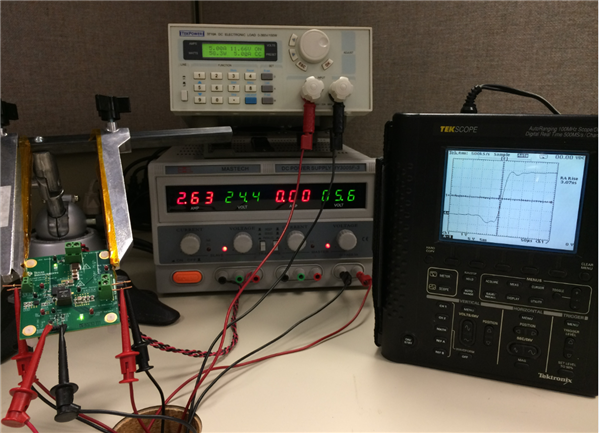# Are you accurately measuring the picosecond rise time of your GaN device?

When measuring picosecond rise time of a gallium nitride (GaN) transistor, even a 1-GHz scope and 1-GHz probe may be inadequate. Accurately measuring a GaN transistor’s rise and fall time necessitates careful attention to your measurement setup and equipment. Let’s walk through the best practices for accurate measurement using TI’s recently introduced LMG5200 integrated half-bridge GaN power module.

Before ordering the LMG5200 evaluation module (EVM), confirm that your bench equipment can accurately measure wide bandgap (WBG) semiconductors like the LMG5200, based on GaN – a technology that the U.S. Department of Energy considers “foundational” in making better use of our energy resources. If your equipment looks like the equipment shown in here, you’ll probably need to upgrade your scope and probes.

Measuring the voltage transition of a WBG semiconductor necessitates equipment with sufficient measurement bandwidth.  The bandwidth of an oscilloscope is characterized by its -3dB frequency – a frequency at which the amplitude of a sinewave (displayed on the oscilloscope) has dropped to 1/√2 of the input signal or 0.707.  In general an oscilloscope will have a 30% amplitude attenuation error if the input signal is equal to the scope’s bandwidth.  If you’re using a Digital Storage Oscilloscope (DSO) the sampling rate imposes other restrictions.  For example, a four channel, 1Gs/sec DSO will typically only have 250Ms/sec capability when all four channels are used and Nyquist sampling requirements apply!   As a rule of thumb, if your scope is rated with a bandwidth of X, then the maximum signal frequency you can faithfully measure (to within 3%) is X/3.  For 1% accuracy your signal should be no greater than X/5.

The oscilloscope probe also adds error to the measurement and can be modeled as a resistor-capacitor (RC) low-pass circuit. What’s more, you’re not looking at a pure sine wave when measuring switching transitions, so what’s the effective bandwidth of a switch node rise and fall time? Mathematically, you can estimate the probe’s output to an applied voltage step as Equation 1:

Vout = Vin(1-e^t/RC)                     (1)

Rise time is most often expressed in terms of an output transition from 10% to 90% of its final value. Using Equation 1, the 10% point is then 0.1RC and the 90% point is 2.3RC and since the time-constant of the probe is 1/2πfRC an expression for bandwidth can be determined.

RC = tr/(t90% - t10%) = tr/2.2RC = 1/2πf                 (2)

Therefore the required bandwidth is given by: bandwidth = 0.35/tr

This relationship allows you to assess a signal’s equivalent bandwidth in terms of rise time. For example, if you expect your GaN device to turn on in 500ps, then you need a scope capable of 0.35/500ps –700MHz – but you also need a probe with at least that much bandwidth. Knowing your probe and scope bandwidth, you can apply a root sum square (RSS) to assess the actual rise time based on the statistical error of the associated measurement equipment as described in this article.

To validate the expected requirements, I evaluated three measurement setups. The first was with a 100MHz/500Ms/sec handheld TekScope; the second was a 500MHz/2.5Gs/sec DPO4051; and the third was a 1GHz/5Gs/sec MDO4104-6. I set up the LMG5200 to convert 24V to 12V at 5A. The switching frequency was set at 1MHz and a duty cycle of 52% was necessary to account for losses in the power stage. I measured the efficiency of the power conversion at ~96%.

To measure the rise time I used the scope cursors. If want the scope to calculate rise time, be sure to set up the sampling rate to capture a sufficient number of data points. Nyquist requires at least two to three sample points, but I’d recommend four or five on the rising edge. This suggests that if the rise time is less than 1ns, even a 5Gs/sec sampling capability would be marginal.

Probe bandwidth is equally important; even my 1GHz probe introduced significant error. Note that in some cases, a scope may have more or less capability than specified depending on the manufacturer, setup calibration, and age of the scope and probes.  From my testing I would say a 1GHz system bandwidth is an absolute minimum requirement for measuring a WBG switch transition.

So what did I find? Using the low-inductive probing technique described in this article, the 100MHz scope shown in Figure 1 measured a rise time of 3ns – clearly an erroneous measurement as can be seen when compared with the 1GHz bandwidth system measurement shown in Figure 2 where the rise time was measured to be 780ps. The 500MHz scope measured a 1ns rise time.Figure 1: 100MHz scope system measuring the LMG5200 switch node while converting 24V to 12V at 1MHz with a rise time equal to 3ns

Figure 2: 1GHz scope system of the LMG5200 switch node converting 24V to 12V at 1MHz with a rise time equal to 780ps

Using the RSS approach discussed earlier, it was calculated that the 780ps measurement is still 29% slower than what could have otherwise been measured with a higher bandwidth system.  To accurately measure the rise time to within 1% requires a system measurement bandwidth of 4GHz!  Assuming this equipment was available, the 780ps rise time I measured would have been 600ps – that’s a 40V/ns slewrate!  In my next post I’ll examine the importance of careful component selection and layout to minimize radiated emissions associated with this kind of dv/dt.